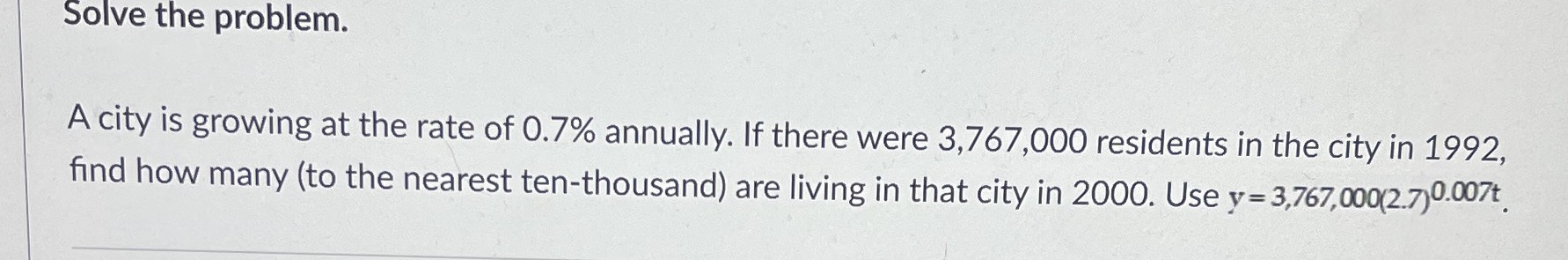### ¿Todavía tienes preguntas de matemáticas?

Pregunte a nuestros tutores expertos
Algebra
PreguntaA city is growing at the rate of $$0.7 \%$$ annually. If there were $$3,767,000$$ residents in the city in $$1992$$ , find how many (to the nearest ten-thousand) are living in that city in $$2000$$ . Use $$y = 3,767,000 ( 2.7 ) ^ { 0.007 t }$$ .